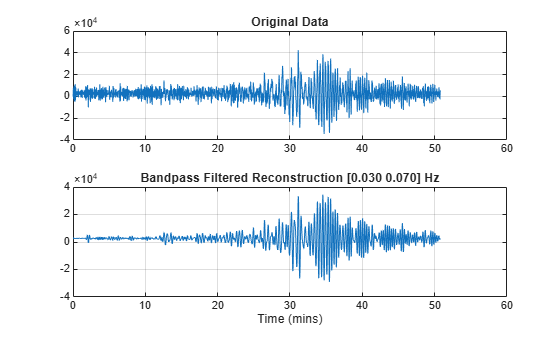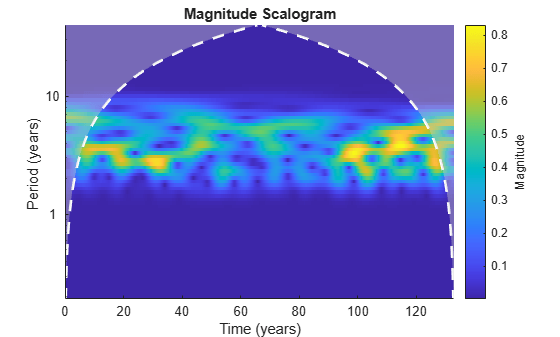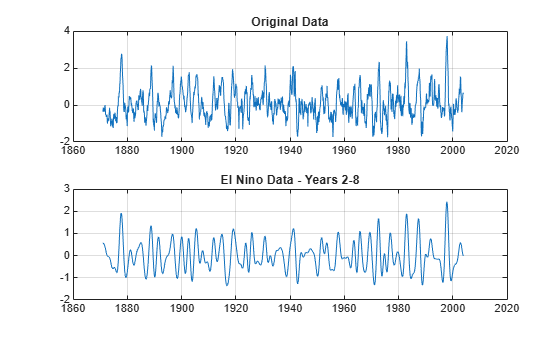Documentation

Frequency- and Time-Localized Reconstruction from the Continuous Wavelet Transform

Reconstruct a frequency-localized approximation of Kobe earthquake data. Extract information from the CWT for frequencies in the range of [0.030, 0.070] Hz.

Obtain the CWT of the data.

[wt,f] = cwt(kobe,1);

Reconstruct the earthquake data, adding the signal mean back into the transformed data.

xrec = icwt(wt,f,[0.030 0.070],'SignalMean',mean(kobe));

Plot and compare the original data and the data for frequencies in the range of [0.030, 0.070] Hz.

subplot(2,1,1)
plot(kobe)
grid on
title('Original Data')
subplot(2,1,2)
plot(xrec)
grid on
title('Bandpass Filtered Reconstruction [0.030 0.070] Hz')You can also use time periods, instead of frequency, with the CWT. Load the El Nino data and obtain its CWT, specifying the time period in years.

[cfs,period] = cwt(nino,years(1/12));

Obtain the inverse CWT for years 2 through 8.

xrec = icwt(cfs,period,[years(2) years(8)]);

Plot the CWT of the reconstructed data. Note the absence of energy outside the band of periods from 2 to 8 years.

figure
cwt(xrec,years(1/12))Compare the original data with the reconstructed data for years 2 through 8.

figure
subplot(2,1,1)
plot(nino)
grid on
title('Original Data')
subplot(2,1,2)
plot(xrec)
grid on
title('El Nino Data - Years 2-8')Wavelet Toolbox DocumentationGet trial now